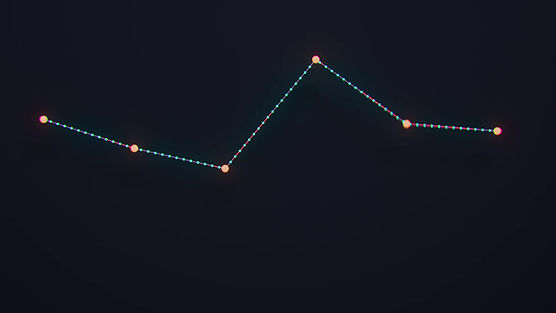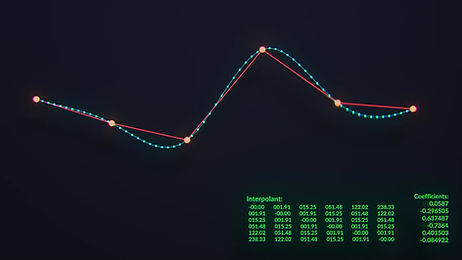### Technical Exploration

Interpolation is the process by which we can estimate, or approximate values given a set of known values.It’s not important to know everything about interpolation to create great-looking visuals, but it can help to understand underlying processes to implement more complicated techniques. Learning about these basic computer graphics topics also helps me to troubleshoot unexpected results. Additionally, Houdini and other graphics processing platforms heavily utilize interpolation in many of its operators and processes. My goal in exploring interpolation is to better understand processing 3d data as a whole.

The resample SOP "exploits" many types of interpolation and is used to generate new point positions that lie between surrounding input points. The key defining factor of interpolation is that it creates new data (new points) based off an arbitrary set of input data (input points/ polyline). Subdivision, smooth, polyreduce, point deform, divide, scatter – these are only a few of the many nodes that also heavily rely on this concept.

Radial Basis Function (RBF) interpolates data based solely on the distances between input data by creating weights associated with values. It is generally more computationally heavy than IDW approximation for large data-sets, but also produces extremely smooth and more visually consistent results, without much tweaking. Additionally it does not produce as much visual artifacting as other methodsrbf interpolation of new input points

point deform interpolation of new input points

RBF also allows for space warping, which effectively deforms the coordinate system of input geometry.  This is because RBF weights every input point regardless of how far or near it is to include in our interpolation solve, whereas IDW only weights points within its distance or max point threshold. Therefore we can think of the resulting interpolation as bending space because every real-valued input point is deformed to a new deformed coordinate-space.

RBF is used extensively in pose-morph deformation weighting as it can create very smooth weighting schemes for arbitrary input data. Each distance is run through a basis function (such as cubic) and inherits a linear sum of weighted deformation values. We can see the weights below by running color through our basis function.

There are many type so interpolation used in computer graphics, but a few basic ones:

Linear interpolation (bilinear and trilinear) – creates a linear function that describes the relationship between two points of data, repeated for the amount of dimensions needed.

Polynomial interpolation (spline) – creates a polynomial function that describes a line that moves through all input data points. Input values can be run through a basis function that can adjust curve profiles (linear, cubic, etc).

IDW approximation – inverse distance weighting; this averages surrounding weighted values relative to input data’s proximity to surrounding pointsrbf interpolation of new input points(space warping)

point deform interpolation of new input pointsrbf weights

rbf weights